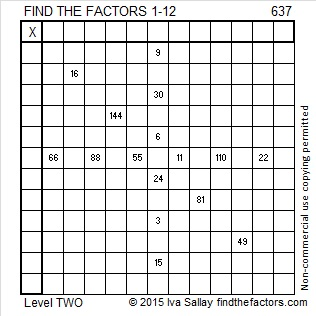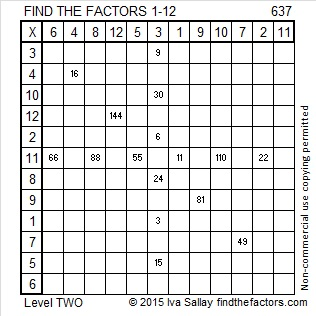# 637 and Level 2

637 is the sum of two perfect squares, 441 and 196, so it is the hypotenuse of a Pythagorean triple, namely 245-588-637. The greatest common factor of those FIVE numbers is also a perfect square. What is it?

637 is the sum of the nineteen prime numbers from 3 to 71.Print the puzzles or type the solution on this excel file: 12 Factors 2015-10-05

—————————————————————————————————

• 637 is a composite number.
• Prime factorization: 637 = 7 x 7 x 13, which can be written 637 = (7^2) x 13
• The exponents in the prime factorization are 2 and 1. Adding one to each and multiplying we get (2 + 1)(1 + 1) = 3 x 2  = 6. Therefore 637 has exactly 6 factors.
• Factors of 637: 1, 7, 13, 49, 91, 637
• Factor pairs: 637 = 1 x 637, 7 x 91, or 13 x 49
• Taking the factor pair with the largest square number factor, we get √637 = (√49)(√13) = 7√13 ≈ 25.2388589.—————————————————————————————————This site uses Akismet to reduce spam. Learn how your comment data is processed.